# The Maths

## Model Calibration

• We generate the unobserved state variable via the transition equation, which is a discrete-time version of the stochastic process in equation (1. We can therefore write the transition equation as
• The Kalman filter is then applied as a recursive procedure to compute the optimal estimator of the state variable at a time t, based on the information at time t and updated continuously as new information becomes available. In order to apply the simple Kalman filter, we assume that both the disturbances and the initial state variable are normally distributed; we can therefore calculate the maximum likelihood function and estimate the model parameter that is otherwise unknown.

# Model Implementation

## More from Ezio Lauro

I am curious about numbers,investments and everything else.

Love podcasts or audiobooks? Learn on the go with our new app.

## Understand the Power of Compounding With This Unofficial Theory and a Simple Math## Expectation & Variance of OLS Estimates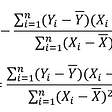## Markov Chain Analysis and Simulation using Python## A Guide to Metrics in Exploratory Data Analysis## System of linear equations | Linear Algebra | Part 4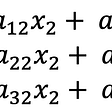## Statistical Power in Hypothesis Testing## Playing infinite chess — 3:16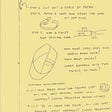## Ezio Lauro

I am curious about numbers,investments and everything else.

## Solving a capacitated vehicle routing problem with Google OR-Tools and Mapbox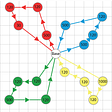## Optimization with Python: Infeasibility Explanation for Integer Programming with OR-Tools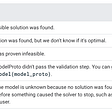## System Reliability Optimization by Evolutionary Strategy